Courses

# RD Sharma Solutions (Part - 1) - Ex-2.1, Fractions, Class 7, Math Class 7 Notes | EduRev

## RD Sharma Solutions for Class 7 Mathematics

Created by: Abhishek Kapoor

## Class 7 : RD Sharma Solutions (Part - 1) - Ex-2.1, Fractions, Class 7, Math Class 7 Notes | EduRev

The document RD Sharma Solutions (Part - 1) - Ex-2.1, Fractions, Class 7, Math Class 7 Notes | EduRev is a part of the Class 7 Course RD Sharma Solutions for Class 7 Mathematics.
All you need of Class 7 at this link: Class 7

#### QUESTION 1:

Compare the following fractions by using the symbol > or < or =:
(i) 7/9 and 8/13
(ii) 11/9 and 5/9
(iii) 37/41 and 19/30
(iv)
17/15 and 119/105

First, we need to find the LCM of denominators in each case. After that, we will equate the denominators in order to compare the two fractions.

(i)
LCM of 9 and 13 is 117.

Now make both fraction equivalent with denominator as 117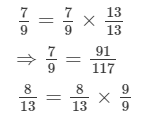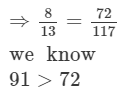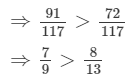(ii)
both fraction have same denominator as 9

we know 11>5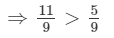(iii)
LCM of 41 and 30 is 1230

Now convert both fraction to their equivalent fractions with denominator as 1230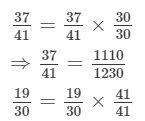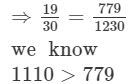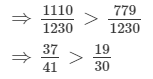(iv)
LCM of 15 and 105 is 105.
Now convert fraction to its equivalent fractions with denominator as 105#### QUESTION 2:

Arrange the following fractions in ascending order: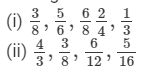(i)
LCM of the denominators 8, 6, 4 and 3 is 24.Now, convert all fractions into their equivalent fractions with denominator 24.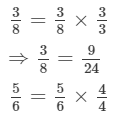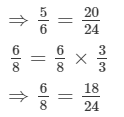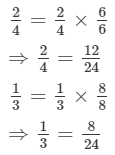we know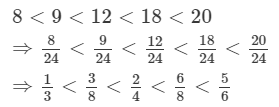(ii)
LCM of the denominators 8, 12, 16 and 3 is 48.Now, convert all fractions into their equivalent fractions with denominator 48.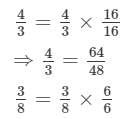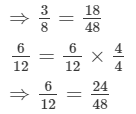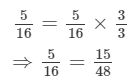we know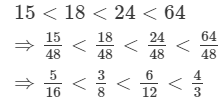#### QUESTION 3:

Arrange the following fractions in descending order: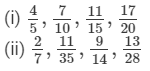(i)
LCM of the denominators 5, 10, 15 and 20 is 60.Now, convert all fractions to their equivalent fractions with denominator 60.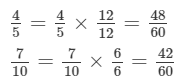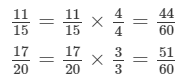we know: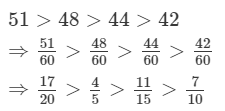(ii)
LCM of the denominators 7, 35, 14 and 28 is 140.Now, convert all fractions to their equivalent fractions with denominator 140.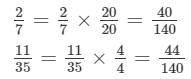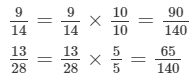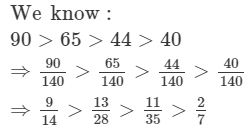#### Question 4:

Write five equivalent fractions of 3/5

Five equivalent fractions of 3/5 are :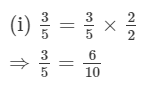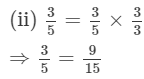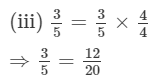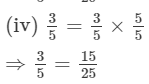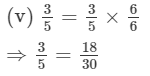#### Question 5:

Find the sum: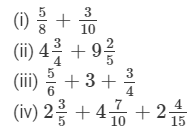(i)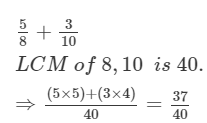(ii)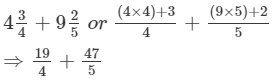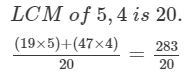(iii)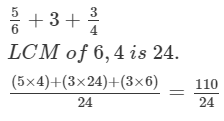(iv)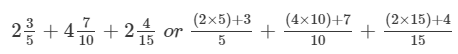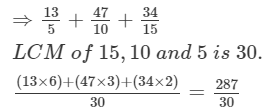#### Question 6:

Find the difference of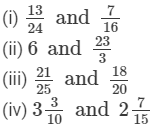(i)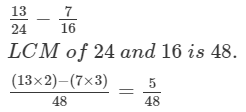(ii)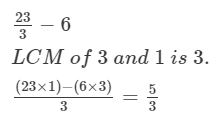(iii)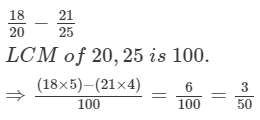(iv)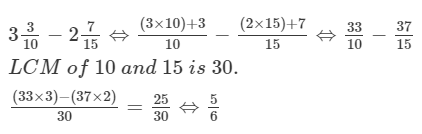#### Question 7:

Find the difference: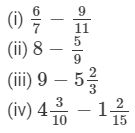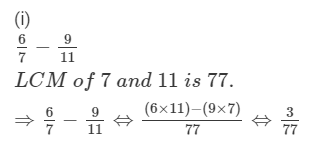(ii)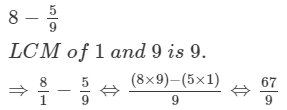(iii)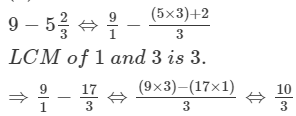#### (iv)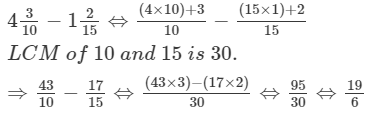#### QUESTION 8: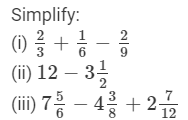(i)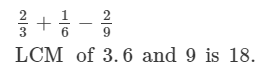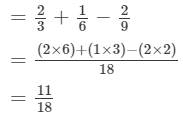(ii)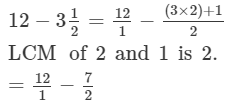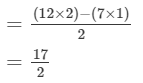(iii)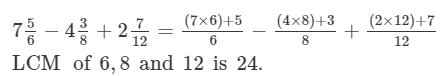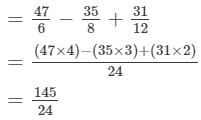#### Question 9:

What should be added to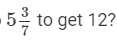Let x be the required fraction.
According to the question: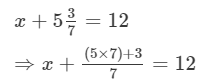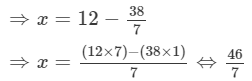97 docs

,

,

,

,

,

,

,

,

,

,

,

,

,

,

,

,

,

,

,

,

,

,

,

,

,

,

,

,

,

,

;